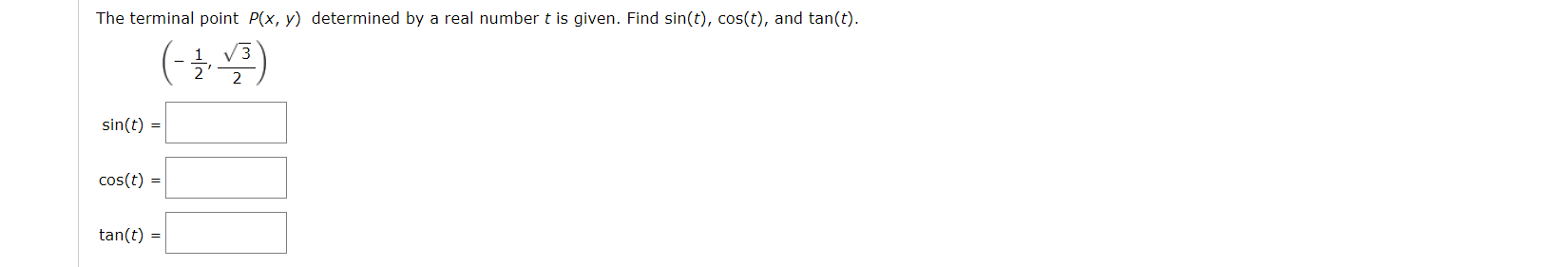# The terminal point P(x, y) determined by a real number t is given. Find sin(t), cos(t), and tan(t).sin(t)cos(t)tan(t)

Questionhelp_outlineImage TranscriptioncloseThe terminal point P(x, y) determined by a real number t is given. Find sin(t), cos(t), and tan(t). sin(t) cos(t) tan(t) fullscreen
check_circleExpert Solution
Step 1

To determine the value of sin(t), cos(t) and tan(t).

Step 2

Given:

The terminal point P(x, y) is determined by a real number t is given:

Step 3

Formula Used:

The polar coordinate for the point (x, y), determined by a re...

### Want to see the full answer?

See Solution

#### Want to see this answer and more?

Solutions are written by subject experts who are available 24/7. Questions are typically answered within 1 hour*

See Solution
*Response times may vary by subject and question
Tagged in

### Other